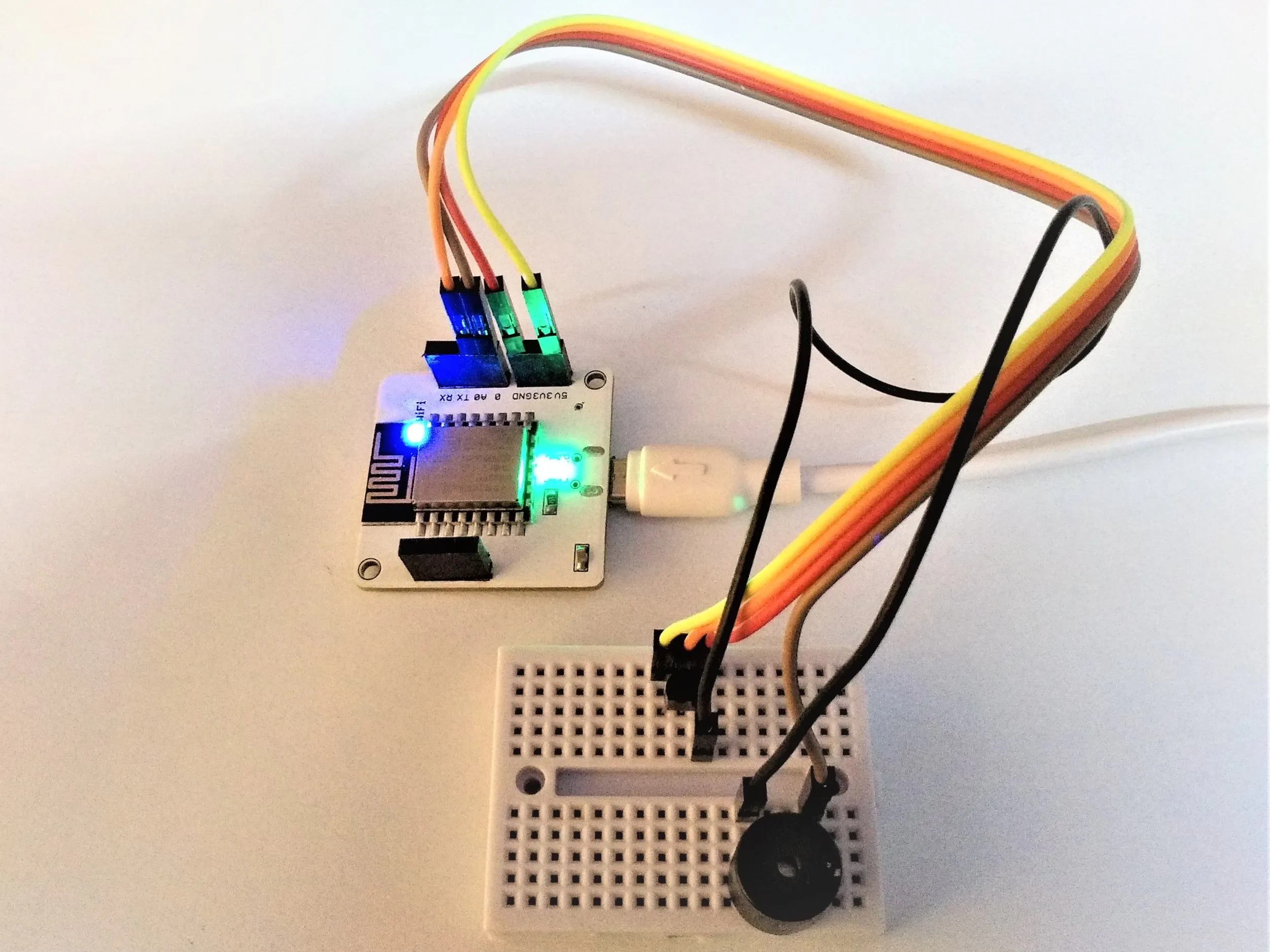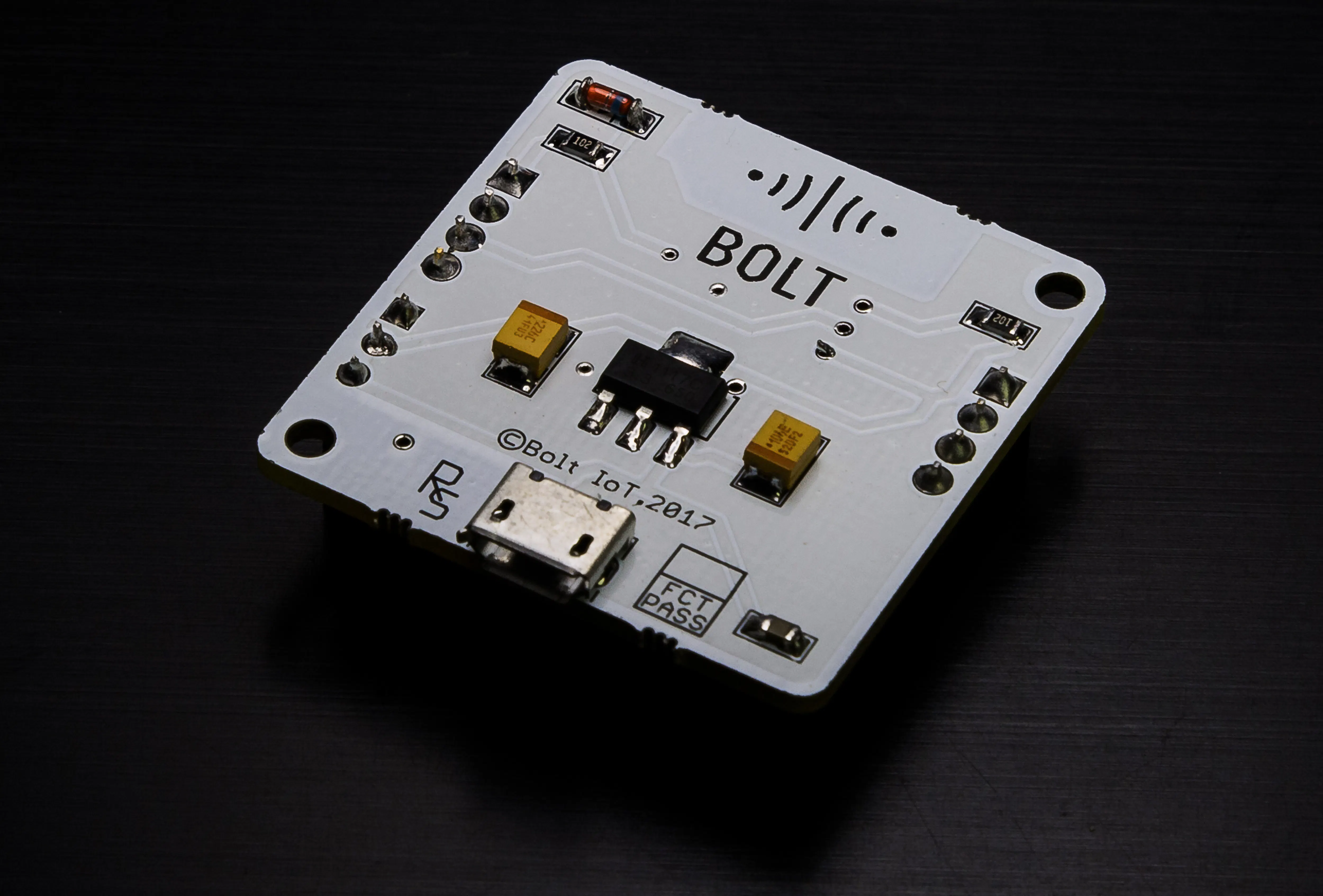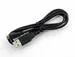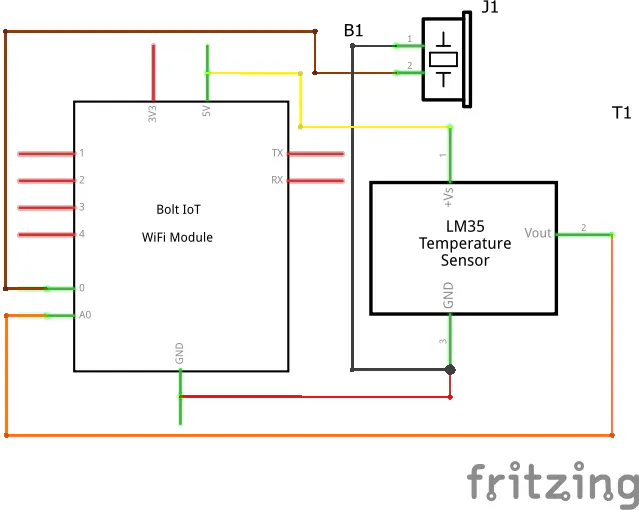# Temperature Monitoring And Two Way Alerting System

The system sends an SMS alert and sounds a buzzer if the temperature crosses certain static thresholds or increases/decreases suddenly.

IntermediateFull instructions provided4 hours277## Things used in this project

### Hardware componentsBolt IoT Bolt WiFi Module
×1USB-A to Micro-USB Cable
×1Temperature Sensor
×1Buzzer
×1Jumper wires (generic)
×5×1

### Software apps and online servicesBolt IoT Bolt CloudTwilio SMS Messaging API
 Ubuntu OS/VPS

## Schematics

### Fritzing Schematic Diagram## Code

Python
Main code with explanation.
```#--------------------------Importing Required Libraries-------------------------

import conf, json, time, math, statistics
from boltiot import Sms, Bolt

#--------------------Defining Function For Z – Score Analysis-------------------

def compute_bounds(history_data, frame_size, factor):
if len(history_data) < frame_size :	#Can’t perform analysis if number of
return None           #previous data points is less than the frame size

if len(history_data) > frame_size :
del history_data[0:len(history_data) - frame_size] #Extra data removed

Mn = statistics.mean(history_data)			               #Calculating mean
Variance = 0
for data in history_data :				              #Loop to calculate variance
Variance += math.pow((data - Mn), 2)
Zn = factor * math.sqrt(Variance / frame_size)	#Calculation of Z – score
High_bound = history_data[frame_size-1] + Zn
Low_bound = history_data[frame_size-1] - Zn
return [High_bound, Low_bound]			   #Dynamic Thresholds stored in a list

response = mybolt.digitalWrite("0", "HIGH")		#Turning ON the buzzer
if data_b["success"]!=1:
print("There was an error while sending a sound alert:", data_b["value"])
time.sleep(30)					                 #Sounding the buzzer for 30 seconds
response = mybolt.digitalWrite("0", "LOW")		#Turning OFF the buzzer

#-------------------------Creating Bolt And SMS Objects-------------------------

mybolt = Bolt(conf.API_KEY, conf.DEVICE_ID)
sms = Sms(conf.SSID, conf.AUTH_TOKEN, conf.TO_NUMBER, conf.FROM_NUMBER)
history_data=[]			#Initializing empty list for storing sensor values

#------Infinite Loop For Collecting Sensor Values, Comparing And Alerting-------

while True:
data = json.loads(response)		      #Convert JSON data to Python dictionary
if data['success']!= 1:
print("There was an error while retrieving the data.")
print("This is the error:", data['message'])
time.sleep(10)					        #Wait for 10 seconds
continue					              #Execute next iteration of while loop

print("The sensor value is:", data['value'])
sensor_value = 0					          #Initializing current sensor value

try:
sensor_value = int(data['value'])
temp = (100 * sensor_value) / 1024			#Converting to Degree Celsius
except e:
print("There was an error while parsing the response:", e)
continue

try:
if sensor_value < conf.min or sensor_value > conf.max:
if sensor_value < conf.min:
print("The sensor value has gone below the lower threshold " + str(conf.min) + ". Sending SMS via Twilio.")
response = sms.send_sms("The temperature at the server room has gone below the lower threshold. The current temperature is " + str(temp) + " Degrees Celsius.")
else:
print("The sensor value has exceeded the higher threshold " + str(conf.max) + ". Sending SMS via Twilio.")
response = sms.send_sms("The temperature at the server room has exceeded the higher threshold. The current temperature is " + str(temp) + " Degrees Celsius.")
print("The response received from Twilio is: "+ str(response))
print("The status of the SMS at Twilio is: " + str(response.status))

except Exception as e:
print("There was an error while sending the SMS:", e)

bound = compute_bounds(history_data, conf.FRAME_SIZE, conf.MUL_FACTOR)
if not bound:
required_data_count = conf.FRAME_SIZE - len(history_data)
print("Not enough data to compute Z-score. Need", required_data_count, "more data points.")
history_data.append(sensor_value) #Current sensor value appended to list
time.sleep(10)
continue

try:
if sensor_value > bound :			   #If value greater than higher bound
print("The temperature increased suddenly. Sending an SMS.")
response = sms.send_sms("The temperature at the server room increased suddenly. The current temperature is " + str(temp) + " Degrees Celsius.")
print("The response received from Twilio is:", response)

elif sensor_value < bound:			    #If value less than lower bound
print("The temperature decreased suddenly. Sending an SMS.")
response = sms.send_sms("The temperature at the server room decreased suddenly. The current temperature is " + str(temp) + " Degrees Celsius.")
print("The response received from Twilio is:", response)
history_data.append(sensor_value)

except Exception as e:
print("Error:", e)

time.sleep(10)			#Collects new sensor values at an interval of 10 seconds
```

## Credits

### Sarita Mandal

1 project • 0 followers
Thanks to Pranav Pai Vernekar.